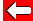The solutions x = 2 and x = -1 to the quadratic equation x² - x - 2 = 0 can reveal something very neat indeed.

We can rearrange x = 2 into x - 2 = 0.

Similarly we can rearrange x = -1 into x + 1 = 0

We can write these as:
(x - 2) = 0 and (x + 1) = 0

If we multiply the bracketed terms, we get: (x - 2)(x + 1) = 0

Multiplying out the brackets: x² - 2x + x - 2 = 0
More neatly: x² - x - 2 = 0. This is our original quadratic equation!

What this means is important: quadratic equations can be factorised, offering a reasonably quick solution.Go back a pageMaths MenuGo to next page